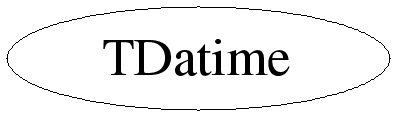# class TDatime

```
TDatime

This class stores the date and time with a precision of one second
in an unsigned 32 bit word (e.g. 950130 124559). The date is stored
with the origin being the 1st january 1995.

This class has no support for time zones. The time is assumed
to be in the local time of the machine where the object was created.
As a result, TDatime objects are not portable between machines
operating in different time zones and unsuitable for storing the
date/time of data taking events and the like. If absolute time is
required, use TTimeStamp.

```

## Function Members (Methods)

public:
 TDatime() TDatime(const TDatime& d) TDatime(const char* sqlDateTime) TDatime(UInt_t tloc, Bool_t dosDate = kFALSE) TDatime(Int_t date, Int_t time) TDatime(Int_t year, Int_t month, Int_t day, Int_t hour, Int_t min, Int_t sec) virtual ~TDatime() const char* AsSQLString() const const char* AsString() const const char* AsString(char* out) const static TClass* Class() UInt_t Convert(Bool_t toGMT = kFALSE) const void Copy(TDatime& datime) const void FillBuffer(char*& buffer) UInt_t Get() const Int_t GetDate() const static void GetDateTime(UInt_t datetime, Int_t& date, Int_t& time) Int_t GetDay() const Int_t GetDayOfWeek() const Int_t GetHour() const Int_t GetMinute() const Int_t GetMonth() const Int_t GetSecond() const Int_t GetTime() const Int_t GetYear() const virtual TClass* IsA() const TDatime& operator=(const TDatime& d) void Print(Option_t* option = "") const void ReadBuffer(char*& buffer) void Set() void Set(const char* sqlDateTime) void Set(UInt_t tloc, Bool_t dosDate = kFALSE) void Set(Int_t date, Int_t time) void Set(Int_t year, Int_t month, Int_t day, Int_t hour, Int_t min, Int_t sec) virtual void ShowMembers(TMemberInspector& insp, char* parent) Int_t Sizeof() const virtual void Streamer(TBuffer& b) void StreamerNVirtual(TBuffer& b)

## Data Members

protected:
 UInt_t fDatime Date (relative to 1995) + time

## Class Charts## Function documentation

TDatime()
``` Create a TDatime and set it to the current time.
```
TDatime(Int_t date, Int_t time)
``` Create a TDatime and set it to the specified date and time.
See Set(Int_t, Int_t) about the date, time format.
```
TDatime(Int_t year, Int_t month, Int_t day, Int_t hour, Int_t min, Int_t sec)
``` Create a TDatime and set it to the specified year, month,
day, time, hour, minute and second. See Set() about the format.
```
TDatime(const char *sqlDateTime)
``` Expects as input a string in SQL date/time compatible format, like:
yyyy-mm-dd hh:mm:ss.
```

``` Returns day of week, with Monday being day 1 and Sunday day 7.
```
const char * AsString(char* out) const
``` Return the date & time as a string (ctime() format).
Copy result because it points to a statically allocated string.
```
const char * AsString(char* out) const
``` Return the date & time as a string (ctime() format).
Result is copied into out (and out is returned). Make sure
out can at least contain 26 characters. Thread safe.
```
const char * AsSQLString()
``` Return the date & time in SQL compatible string format, like:
1997-01-15 20:16:28. The returned string buffer is static and
will be reused.
```
UInt_t Convert(Bool_t toGMT = kFALSE) const
``` Convert fDatime from TDatime format to the standard time_t format.
If toGMT is true, the time offset of the current local time zone is
subtracted from the returned time_t. One use of such a non-standard time_t
value is to convert a TDatime object that contains local time to GMT,
as in this example:

TDatime now;
now.Set(now.Convert(kTRUE));

Caution: the time_t returned from Convert(kTRUE) is incompatible with
regular Unix time - it contains an artificial, locale-dependent offset.
```
void Copy(TDatime& datime) const
``` Copy this to datime.
```
void FillBuffer(char*& buffer)
``` Encode Date/Time into buffer, used by I/O system.
```

``` Return date in form of 19971224 (i.e. 24/12/1997)
```

``` Return time in form of 123623 (i.e. 12:36:23)
```
void Print(Option_t* option = "") const
``` Print date and time.
```
``` Decode Date/Time from output buffer, used by I/O system.
```
void Set(const char* sqlDateTime)
``` Set Date/Time to current time as reported by the system.
Date and Time are encoded into one single unsigned 32 bit word.
Date is stored with the origin being the 1st january 1995.
Time has 1 second precision.
```
void Set(UInt_t tloc, Bool_t dosDate)
``` The input arg is a time_t value returned by time() or a value
returned by Convert(). This value is the number of seconds since
the EPOCH (i.e. 00:00:00 on Jan 1m 1970). If dosDate is true then
the input is a dosDate value.
```
void Set(Int_t date, Int_t time)
``` Set date and time. Data must be in format 980418 or 19980418 and time in
224512 (second precision). The date must
be >= 950101.
For years >= 2000, date can be given in the form 20001127 or 1001127
internally the date will be converted to 1001127
```
void Set(Int_t year, Int_t month, Int_t day, Int_t hour, Int_t min, Int_t sec)
``` Set date and time. Year may be xx where 95 <= xx <= 199 (199 being 2099).
The year must be >= 1995.
```
void Set(const char* sqlDateTime)
``` Expects as input a string in SQL date/time compatible format, like:
yyyy-mm-dd hh:mm:ss.
```
void Streamer(TBuffer& b)
``` Stream a object of type TDatime.
```
void GetDateTime(UInt_t datetime, Int_t& date, Int_t& time)
``` Static function that returns the date and time. The input is
in TDatime format (as obtained via TDatime::Get()).
Date is returned in the format 950223  February 23 1995.
Time is returned in the format 102459 10h 24m 59s.
```
TDatime& operator=(const TDatime& d)
`{ fDatime = d.fDatime; return *this; }`
TDatime()
TDatime(const TDatime &d)
`{ }`
virtual ~TDatime()
`{ }`

`{ return fDatime; }`

`{ return (fDatime>>26) + 1995; }`

`{ return (fDatime<<6)>>28; }`

`{ return (fDatime<<10)>>27; }`

`{ return (fDatime<<15)>>27; }`

`{ return (fDatime<<20)>>26; }`

`{ return (fDatime<<26)>>26; }`

`{return sizeof(UInt_t);}`

Author: Rene Brun 05/01/95
Last update: root/base:\$Id: TDatime.h 20877 2007-11-19 11:17:07Z rdm \$